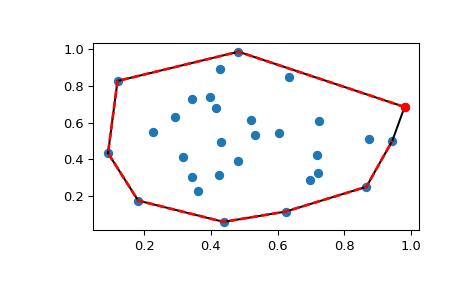# scipy.spatial.ConvexHull¶

class scipy.spatial.ConvexHull(points, incremental=False, qhull_options=None)

Convex hulls in N dimensions.

New in version 0.12.0.

Parameters: points : ndarray of floats, shape (npoints, ndim) Coordinates of points to construct a convex hull from incremental : bool, optional Allow adding new points incrementally. This takes up some additional resources. qhull_options : str, optional Additional options to pass to Qhull. See Qhull manual for details. (Default: “Qx” for ndim > 4 and “” otherwise) Option “Qt” is always enabled. QhullError Raised when Qhull encounters an error condition, such as geometrical degeneracy when options to resolve are not enabled. ValueError Raised if an incompatible array is given as input.

Notes

The convex hull is computed using the Qhull library.

References

Examples

Convex hull of a random set of points:

>>> from scipy.spatial import ConvexHull
>>> points = np.random.rand(30, 2)   # 30 random points in 2-D
>>> hull = ConvexHull(points)


Plot it:

>>> import matplotlib.pyplot as plt
>>> plt.plot(points[:,0], points[:,1], 'o')
>>> for simplex in hull.simplices:
...     plt.plot(points[simplex, 0], points[simplex, 1], 'k-')


We could also have directly used the vertices of the hull, which for 2-D are guaranteed to be in counterclockwise order:

>>> plt.plot(points[hull.vertices,0], points[hull.vertices,1], 'r--', lw=2)
>>> plt.plot(points[hull.vertices,0], points[hull.vertices,1], 'ro')
>>> plt.show()Attributes

 points (ndarray of double, shape (npoints, ndim)) Coordinates of input points. vertices (ndarray of ints, shape (nvertices,)) Indices of points forming the vertices of the convex hull. For 2-D convex hulls, the vertices are in counterclockwise order. For other dimensions, they are in input order. simplices (ndarray of ints, shape (nfacet, ndim)) Indices of points forming the simplical facets of the convex hull. neighbors (ndarray of ints, shape (nfacet, ndim)) Indices of neighbor facets for each facet. The kth neighbor is opposite to the kth vertex. -1 denotes no neighbor. equations (ndarray of double, shape (nfacet, ndim+1)) [normal, offset] forming the hyperplane equation of the facet (see Qhull documentation for more). coplanar (ndarray of int, shape (ncoplanar, 3)) Indices of coplanar points and the corresponding indices of the nearest facets and nearest vertex indices. Coplanar points are input points which were not included in the triangulation due to numerical precision issues. If option “Qc” is not specified, this list is not computed. area (float) Area of the convex hull volume (float) Volume of the convex hull

Methods

 add_points(points[, restart]) Process a set of additional new points. close() Finish incremental processing.